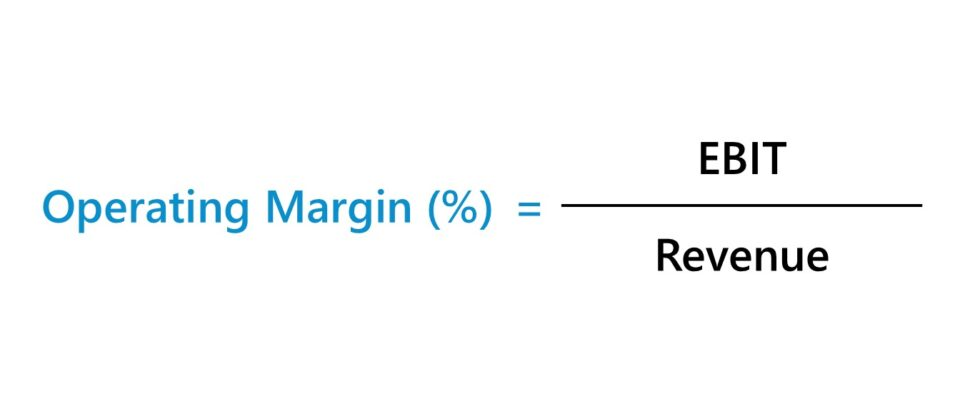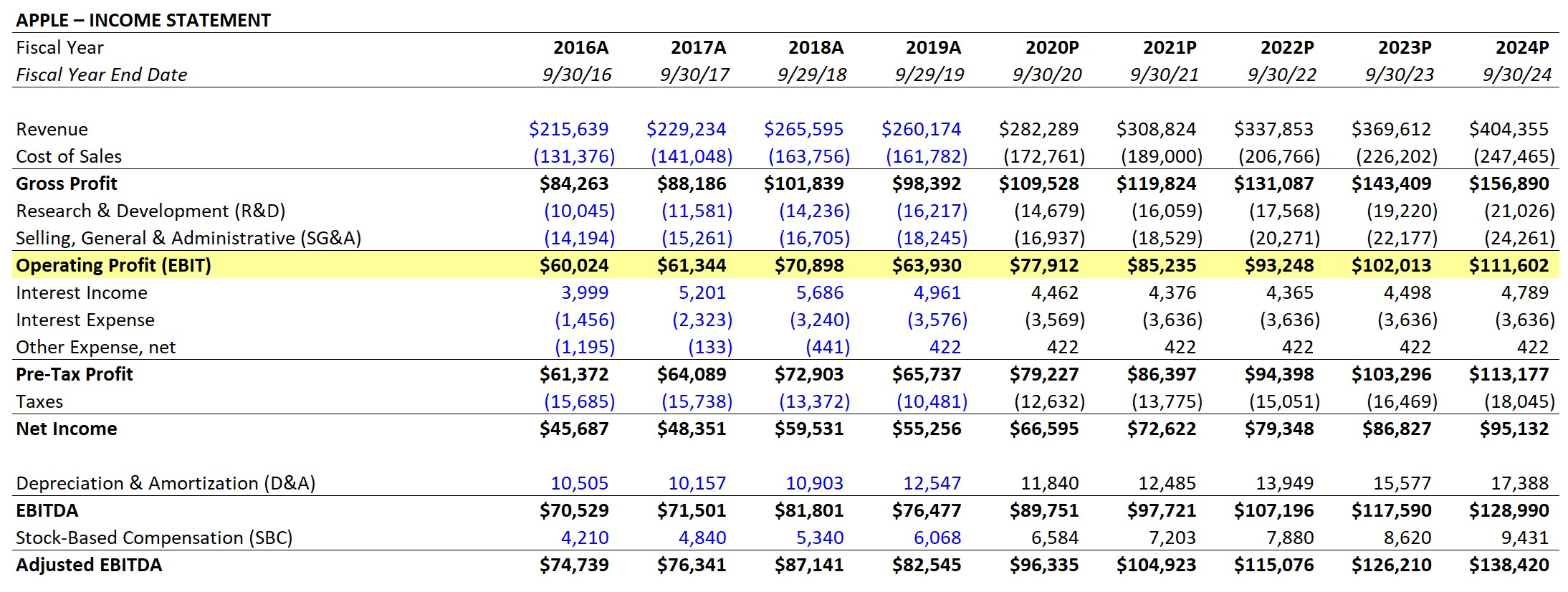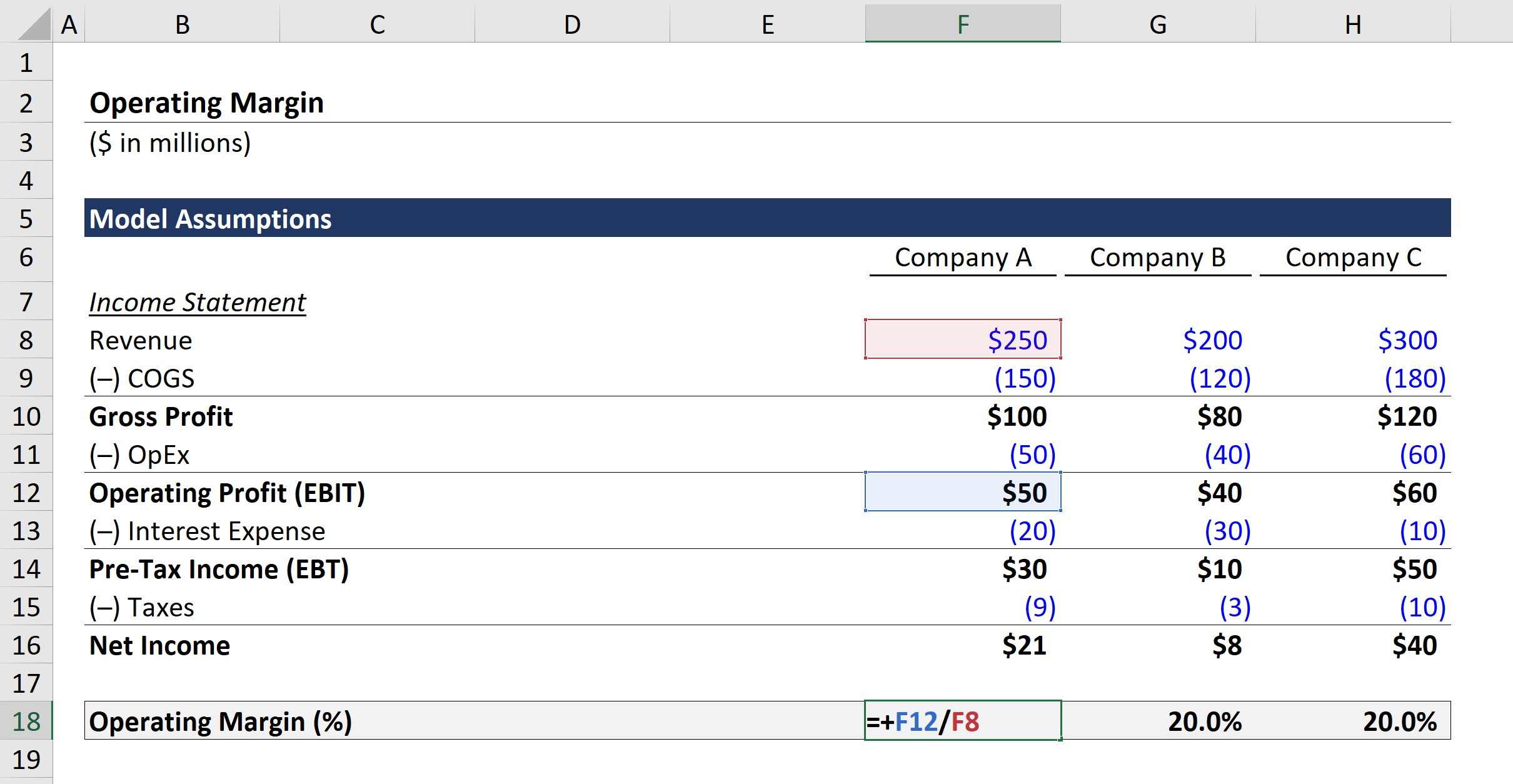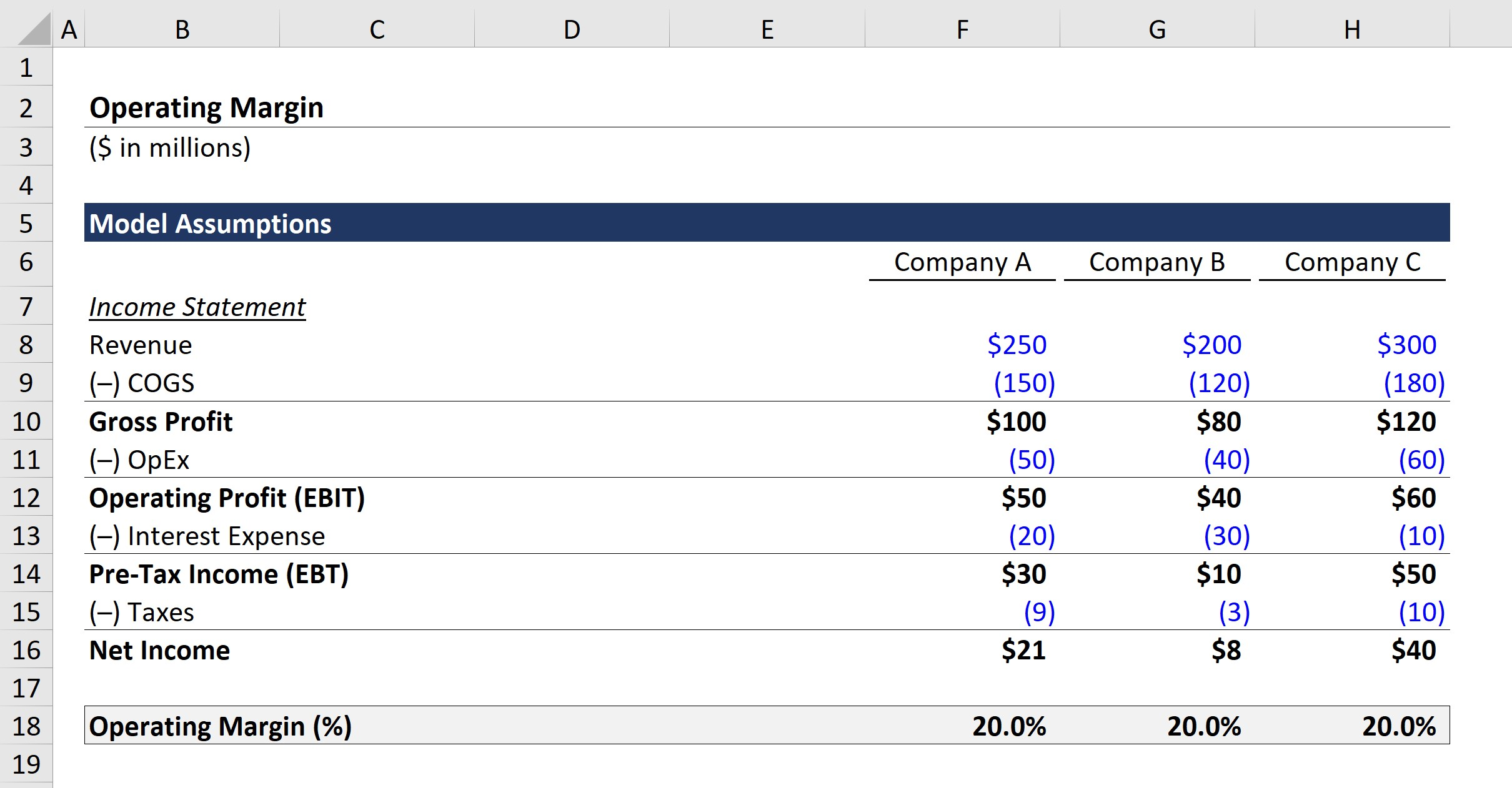# Operating Margin

Guide to Understanding Operating Margin (%)## How to Calculate Operating Margin (Step-by-Step)

The operating margin is the ratio between a company’s operating profit (i.e. EBIT) and revenue, expressed as a percentage.

The operating profit margin, often referred to as “operating margin,” answers the question, “For each dollar of revenue generated, how much trickles down to operating income (EBIT)?”

The operating income of a company can be calculated by subtracting operating expenses (OpEx) from gross profit, which has already been reduced by the cost of goods sold (COGS) in the period.

With that said, operating income is the remaining earnings once all expenses related to core operations are accounted for (i.e. inclusive of both COGS and OpEx).

For a given period, the accrual accounting-based revenue and operating income of a company can be found on the income statement.

Revenue to EBIT Bridge Description
Revenue
• Net Sales Generated from Selling Products and/or Services to Customers
Cost of Goods Sold (COGS)
• Costs Directly Associated with the Company’s Core Revenue Model
Gross Profit
• Gross Profit = Revenue – COGS
Operating Expenses (SG&A)
• Indirect Costs NOT Directly Related to the Company’s Revenue Model
Operating Income (EBIT)
• Operating Income = Gross Profit – Operating Expenses

The COGS line item refers to direct costs such as materials and direct labor, while OpEx consists of expenses related to selling, general & administrative (SG&A) expenses, research & development (R&D) expenses, and more.

The calculation process is as follows:

• Step 1: First, we must find out each company’s revenue, cost of goods sold (COGS), and operating expenses (OpEx) from their income statements.
• Step 2: Next, we’ll calculate the operating income (EBIT) by subtracting OpEx from gross profit.
• Step 3: Lastly, we’ll divide the operating income of each company by the corresponding revenue amount to arrive at the operating profit margin.

## Operating Margin Formula

The operating profit margin formula consists of dividing a company’s operating income (i.e. EBIT) by the revenue generated in the same period, as shown below.

Operating Margin = EBIT ÷ Revenue

To facilitate comparisons across historical periods as well as against industry peers, the operating profit margin is denoted in percentage form, which is achieved by multiplying the value in decimal form by 100.

## Operating Margin Calculation Example (%)

For example, if a company has generated \$10 million in revenue with \$4 million in COGS and \$2 million in operating expenses (SG&A), the operating profit is \$4 million.

To arrive at the operating profit margin, we’ll divide the \$4 million in EBIT by the \$10 million in revenue and multiply by 100, which comes out to an operating profit margin of 40%.

• Operating Margin (%) = \$4 million ÷ \$10 million = .40, or 40%

In this case, the company earns \$0.40 in operating income for each \$1.00 of revenue generated.

Another way to interpret the \$0.40 is that for each \$1.00 of revenue generated, the company has \$0.40 left to cover non-operating expenses.

### The Wharton Online and Wall Street Prep Private Equity Certificate Program

Level up your career with the world's most recognized private equity investing program. Enrollment is open for the Sep. 5 - Oct. 27 cohort.

## Operating Profit Margin Example: Apple (AAPL) Income Statement

The historical income statement for Apple (AAPL) can be found below, with the operating profit (EBIT) line item highlighted.

######Apple Operating Profit (Source: WSP Financial Statement Modeling)

Given the operating profit and revenue figures for 2019, the operating profit margin comes out to 24.6%.

• Operating Profit Margin (%) = \$63,930 ÷ \$260,174 = 0.246, or 24.6%

Note: The income statement of Apple is presented in units of thousands.

## How to Interpret Operating Margin by Industry (High vs. Low)

### What is a Good Operating Margin?

For comparisons of operating profit margins to be practical, the companies selected should ideally operate in the same (or adjacent) industries, since there are industry-specific factors that can affect the operating profit margin metric.

• Higher EBIT Margin → In general, higher margins are more favorable relative to lower margins, since this means that the company is creating more profits from its operations to pay for its variable costs and fixed costs. High margins relative to the industry average, or margins trending upward across time, demonstrate management’s efficiency at increasing operating profits.
• Lower EBIT Margin → On the other hand, low or declining EBIT margins could be a sign of underlying weaknesses in the company’s future trajectory caused by ineffective growth strategies and inefficient capital allocation.

## Operating Profit Margin vs. Gross Margin vs. Net Margin

The operating profit (EBIT) line item on the income statement separates the operating and non-operating line items.

By definition, earnings before interest and taxes (EBIT) exclude non-operating income and expenses, which are said to be “below the line.”

The non-operating section could be comprised of non-operating activities and one-time gains/(losses), such as interest income from marketable securities, gains on the sale of assets, impairment related to assets like inventory, and income taxes.

In effect, the operating profit margin can be viewed as being the middle ground between the gross profit margin and net profit margin, as the gross margin only accounts for direct costs (i.e. COGS) whereas the net profit margin accounts for all operating expenses and non-operating expenses.

The effects of certain discretionary financing decisions, accounting policies, and tax structures are neglected from the EBIT margin, which allows the metric to be more informative for peer comparisons.

## Limitations of EBIT Margin (%)

• Non-Cash Items (D&A): One frequent area of criticism for the EBIT margin is that the metric includes non-cash expenses such as depreciation and amortization (D&A) and stock-based compensation.
• Capital Expenditure (Capex): The EBIT profit metric also fails to account for capital expenditures (Capex), which represent a recurring outflow of cash and the most significant expenditure for most, if not all, companies. Thus, considering the sheer magnitude of the impact that a company’s maintenance and growth capital expenditures (Capex) could have on the actual free cash flows (FCFs) and liquidity of a company, neglecting the line item is a common source of criticism.

## Operating Margin Calculator – Excel Template

We’ll now move to a modeling exercise, which you can access by filling out the form below.Submitting ...

## Step 1. Financial Assumptions of Peer Group

Suppose we’re tasked with calculating and comparing the operating profit margins of three companies that are all close competitors operating in the same industry.

The peer group also has relatively similar financial profiles related to their “core” operations.

We’ll first list the financial assumptions related to the three major inputs, which are revenue, cost of goods sold, and operating expenses.

Company A, Model Assumptions:

• Revenue = \$250m
• Cost of Goods Sold (COGS) = –\$150m
• Operating Expenses (OpEx) = –\$50m

Company B, Model Assumptions:

• Revenue = \$200m
• Cost of Goods Sold (COGS) = –\$120m
• Operating Expenses (OpEx) = –\$40m

Company C, Model Assumptions:

• Revenue = \$300m
• Cost of Goods Sold (COGS) = –\$180m
• Operating Expenses (OpEx) = –\$60m

## Step 2. Operating Income Calculation Example (EBIT)

Using the provided assumptions, we can calculate the operating profit (EBIT) for each company by subtracting OpEx from gross profit.

Operating Profit (EBIT):

• Company A = \$100m – \$50m = \$50m
• Company B = \$80m – \$40m = \$40m
• Company C = \$120m – \$60m = \$60m

## Step 3. Operating Profit Margin Calculation Example

In the next step, the operating profit margins for each company can be calculated by dividing EBIT by revenue.

Operating Margin (%):

• Company A = \$50m ÷ \$250m = 20.0%
• Company B = \$40m ÷ \$200m = 20.0%
• Company C = \$60m ÷ \$300m = 20.0%## Step 4. Operating Margin Ratio Analysis

The operating profit margin for all three companies comes out to 20.0%, but as we can see from the expenses “below the line”, the companies have made different financing decisions, as implied by the different interest expense amounts.

The effects of the differences in financing as well as the different tax rates are not incorporated into the operating margin metric, which allows EBIT to be among the most widely used profitability measures for comparison purposes.Step-by-Step Online Course

### Everything You Need To Master Financial Modeling

Enroll in The Premium Package: Learn Financial Statement Modeling, DCF, M&A, LBO and Comps. The same training program used at top investment banks.Inline FeedbacksLearn Financial Modeling Online

Everything you need to master financial and valuation modeling: 3-Statement Modeling, DCF, Comps, M&A and LBO.

X

The Wall Street Prep Quicklesson Series

7 Free Financial Modeling Lessons

Get instant access to video lessons taught by experienced investment bankers. Learn financial statement modeling, DCF, M&A, LBO, Comps and Excel shortcuts.